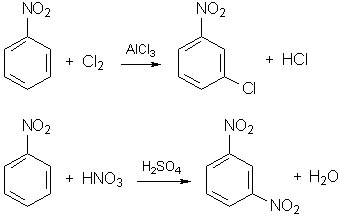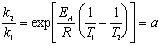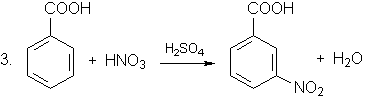# Solutions of the problems for entrance examinations

## Main round

Problem 1.

The required substance is H2S.

H2S + 2FeCl3 = S + 2FeCl2 + 2HCl

H2S + 2AgNO3 = Ag2S+ 2HNO3

Problem 2.

The methyl radical - CH3 displays +I-effect and, hence, increases the activity of aromatic system in electrophilic substitution compared to that in benzene. The chlorine atom displays both ñI and +M-effects; it reduces the activity of aromatic system, but this reduction is not as strong as in the case of nitro group - NO2, which displays only negative (ñM) electronic effect.

So, the required list is: toluene > chlorobenzene > nitrobenzene.

The reactions are (nitro group - NO2 directs the substitution into meta-position):Problem 3.

To solve the problem, we should express the ratio of rate constants at temperatures T2 and T1 using the Arrhenius equation. For the first reaction:.

Activation energy of the second reaction is half of that of the first reaction: EA' = EA / 2; hence, the ratio for the second reaction is:.

Problem 4.

X - CH3, Y - COOH.

The reactions:(5C6H5CH3 + 6KMnO4 + 9H2SO4 = 5C6H5COOH + 3K2SO4 + 6MnSO4 + 14H2O).Problem 5.

P2O3 + 2H2SO4(conc) + H2O = 2H3PO4 + 2SO2 (P+3S+4)

SO2 + Ba(OH)2 = BaSO3+ H2O (S+4Ba+2)

BaSO3 + 3C = BaS + 3CO (upon heating) (Ba+2C+2).

Problem 6.

HCOOH + KOH = HCOOK + H2O

CH3COOH + KOH = CH3COOK + H2O

HCOOK + Ag2O2AgH2CO + 2Ag2O4AgLet(HCOOH) = x,(CH3COOH) = y,(H2CO) = z. It follows from reactions with KOH that(KOH) = x + y = 18.7. 1.07. 0.084 / 56 = 0.03 mol.

It follows from reactions with Ag2O that(Ag2O) = 2x + 4z = 9.72 / 108 = 0.09 mol.

The mass of the initial solution is: 46x + 60y + 30z = 2.33 g.

Solving the system of three linear equations, we find: x = 0.005, y = 0.025, z = 0.02.

The molar fractions of the components are: 10% HCOOH, 50% CH3COOH, 40% H2CO.

Problem 7.(Cu) = 4.8 / 64 = 0.075 mol;(NaOH) = 100. 0.1 / 40 = 0.25 mol.

 0.075 0.15 Cu + 4HNO3 = Cu(NO3)2 + 2NO2 + 2H2O
 0.15 0.075 0.075 2NO2 + 2NaOH = NaNO2 + NaNO3 + H2O (NaOH is an excess)

The maximum amount of iodine can be obtained through complete reduction of nitrite and nitrate ions to NO:

 0.075 0.0375 2NO2ñ + 2Iñ + 4H+ = I2 + 2NO + 2H2O
 0.075 0.1125 2NO3ñ + 6Iñ + 8H+ = 3I2 + 2NO + 4H2O(I2) = 0.0375 + 0.1125 = 0.15 mol, m(I2) = 0.15. 254 = 38.1 g.